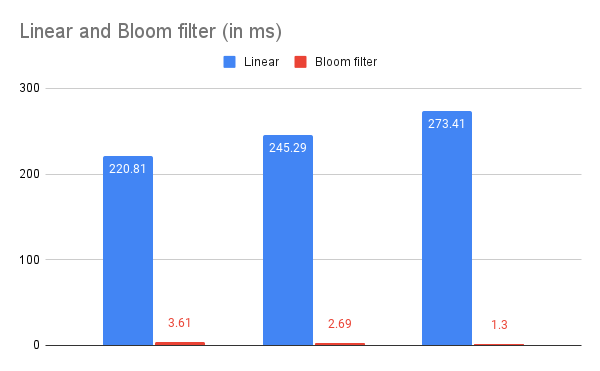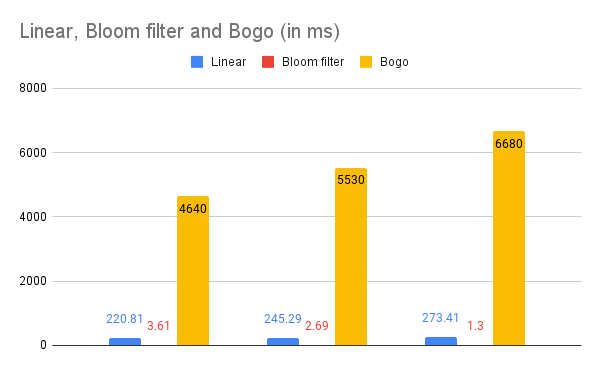## Intro

A bloom filter is a data structure that allows you to quickly identify if some data has been previously added to the structure. What makes a bloom filter unique is that is that it gives up full accuracy for huge speed boost. A bloom filter has small false positive rate, and this rate can be decreased by using more memory and more hash algorithms, however you can find an optimal amount of memory and hash algorithm count to achieve great speed while still maintaining lower memory than a normal list. This specific implementation uses three different hashing algorithms.

## Use cases

Bloom filters are very convenient for many different use cases.

My favorite application is for checking if a username or unique id exists somewhere. Bloom filters have very low memory usage as well as being fast, so for a solution that doesn’t need 100% accuracy and can get away with something close to 99%, then a bloom filter might be the correct structure.

## Implementation

We will define a structure in Rust to represent the bloom filter.

struct BloomFilter {
size: usize,
hash_count: i8,
bitvector: BitVec,
}


Bloom filters usually have two traits (methods) associated with the structure.

1. add an item to the structure
fn add(&mut self, value: String);

1. check if an item likely exists in the structure
fn check(&self, value: String) -> bool;


We define these traits for the structure by “Implementing them like this”.

trait Filter {
fn check(&self, value: String) -> bool;
fn hash(&self, s: String, i: usize) -> i32;
}

impl Filter for BloomFilter {
fn add(&mut self, value: String) {
// ...
}

fn check(&self, value: String) -> bool {
// ...
}

fn hash(&self, s: String, i: usize) -> i32 {
// ...
}
}


For the add trait, we need to call each hash function for the value given to get a likely unique set of keys for the value.

fn add(&mut self, value: String) {
for x in 0..self.hash_count {
let v = self.hash(value.clone(), x.try_into().unwrap());
let k = v as usize % self.size;

self.bitvector.set(k, true);
}
}


We need to do something similar to check if a value has been added.

fn check(&self, value: String) -> bool {
let mut acc = 0;
for x in 0..self.hash_count {
let v = self.hash(value.clone(), x.try_into().unwrap());
let k = v as usize % self.size;

if self.bitvector.get(k).unwrap_or(false) {
acc += 1;
}
}
return acc >= self.hash_count;
}


The hash function is just a collection of the other hash functions.

fn hash(&self, s: String, i: usize) -> i32 {
let functions: [&dyn Fn(String) -> i32; 3] = [&hash_1, &hash_2, &hash_3];
return functions[i](s);
}


Here are the other hash functions.

fn hash_1(s: String) -> i32 {
let mut hash = 0;
let size = s.len();

for i in 0..size {
hash = hash + (s.chars().nth(i)).unwrap() as i32 - 0x30;
}
hash
}

fn hash_2(s: String) -> i32 {
let mut hash = 7;
let size = s.len();

for i in 0..size {
hash = (hash * 31 + (s.chars().nth(i)).unwrap() as i32 - 0x30) % size as i32;
}
hash % size as i32
}

fn hash_3(s: String) -> i32 {
(hash_2(s) + 7) * 3
}


All together, it should look like this.

fn hash_1(s: String) -> i32 {
let mut hash = 0;
let size = s.len();

for i in 0..size {
hash = hash + (s.chars().nth(i)).unwrap() as i32 - 0x30;
}
hash
}

fn hash_2(s: String) -> i32 {
let mut hash = 7;
let size = s.len();

for i in 0..size {
hash = (hash * 31 + (s.chars().nth(i)).unwrap() as i32 - 0x30) % size as i32;
}
hash % size as i32
}

fn hash_3(s: String) -> i32 {
(hash_2(s) + 7) * 3
}

struct BloomFilter {
size: usize,
hash_count: i8,
bitvector: BitVec,
}

trait Filter {
fn check(&self, value: String) -> bool;
fn hash(&self, s: String, i: usize) -> i32;
}

impl Filter for BloomFilter {
fn add(&mut self, value: String) {
for x in 0..self.hash_count {
let v = self.hash(value.clone(), x.try_into().unwrap());
let k = v as usize % self.size;

self.bitvector.set(k, true);
}
}

fn check(&self, value: String) -> bool {
let mut acc = 0;
for x in 0..self.hash_count {
let v = self.hash(value.clone(), x.try_into().unwrap());
let k = v as usize % self.size;

if self.bitvector.get(k).unwrap_or(false) {
acc += 1;
}
}
return acc >= self.hash_count;
}

fn hash(&self, s: String, i: usize) -> i32 {
let functions: [&dyn Fn(String) -> i32; 3] = [&hash_1, &hash_2, &hash_3];
return functions[i](s);
}
}


## Other types of searches

For testing purposes, we can use two different types of searches to compare against the bloom filter.

Linear search iterates through the array, checking if it exists. This has a linear time complexity O(n) and is not ideal for this and many other use cases.

/// Search for the term using linear search
fn linear_search(array: &[String], term: String) -> bool {
for c in array {
if c == term.as_str() {
return true;
}
}
return false;
}


Bogo search is an algorithm that was designed to be purposefully bad. This algorithm has a time complexity of factorial time O(n!). This algorithm should never ever be used.

It essentially picks a random number to use at an index to check if the item is at that index. If it’s not, it repeats.

/// Search for the term using the worst search algorithm, bogo search
fn bogo_search(array: &[String], term: String) -> bool {
let mut num: usize;
loop {
if array[num] == term.as_str() {
return true;
}
}
}


## Testing and Setup

The rest of the code in the project is for setting up the data to be searched and testing of the search algorithms.

fn fill_array_and_bloom_filter(num_vec: &mut [String], bf: &mut BloomFilter) -> Result<()> {
let file = File::open("english-words/words.txt")?;

let mut index = 0;
if index < num_vec.len() {
let l = line?;
num_vec[index] = l.clone();
// Add word to bloom filter
}
index += 1;
}

Ok(())
}

fn main() {
// Set up bloom filter
let mut bf = BloomFilter {
bitvector: BitVec::from_elem(10000, false),
hash_count: 3,
size: 10000,
};

// Set up num vec
let mut num_vec: Vec<String> = vec![String::new(); 17000];

fill_array_and_bloom_filter(&mut num_vec, &mut bf).unwrap();

let length = num_vec.len();

// Pick random term
let x: usize = rng.gen_range(0..num_vec.len());
let term: String = num_vec[x].clone();
println!("The randomly selected term is '{term}'");

// Calculate all of the hashes for the term
let one = hash_1(term.clone());
let two = hash_2(term.clone());
let three = hash_3(term.clone());
println!("The hashes for '{term}' are {one}, {two}, {three}\n");

println!(
"This will test how fast the word '{term}' can be found in the array of {length} words."
);

// Test the time it takes for linear search
let before = Instant::now();
for _ in 0..100 {
linear_search(&mut num_vec, term.clone());
}
println!("Elapsed time for linear_search: {:.2?}", before.elapsed());

// Test the time it takes for bloom filter check
let before = Instant::now();
for _ in 0..100 {
bf.check(term.clone());
}
println!(
"Elapsed time for bloom filter check: {:.2?}",
before.elapsed()
);

// Test the time is takes for bogo search
let before = Instant::now();
for _ in 0..100 {
bogo_search(&mut num_vec, term.clone());
}
println!("Elapsed time for bogo_search: {:.2?}", before.elapsed());
}


## Results

The randomly selected term is 'amicabilities'
The hashes for 'amicabilities' are 736, 11, 54

This will test how fast the word 'amicabilities' can be found in the array of 17000 words.
Elapsed time for linear_search: 220.81ms
Elapsed time for bloom filter check: 3.61ms
Elapsed time for bogo_search: 4.64s

The randomly selected term is 'Aldermaston'
The hashes for 'Aldermaston' are 618, 0, 21

This will test how fast the word 'Aldermaston' can be found in the array of 17000 words.
Elapsed time for linear_search: 245.29ms
Elapsed time for bloom filter check: 2.69ms
Elapsed time for bogo_search: 5.53s

The randomly selected term is 'Achille'
The hashes for 'Achille' are 354, 1, 24

This will test how fast the word 'Achille' can be found in the array of 17000 words.
Elapsed time for linear_search: 273.41ms
Elapsed time for bloom filter check: 1.30ms
Elapsed time for bogo_search: 6.68s## Setting up the project

### Cloning

git clone https://github.com/JakeRoggenbuck/bloom-filter-rs.git
cd bloom-filter-rs


### Words Submodule

git submodule init
git submodule update